# How to Count Times Between Given Time Range in Excel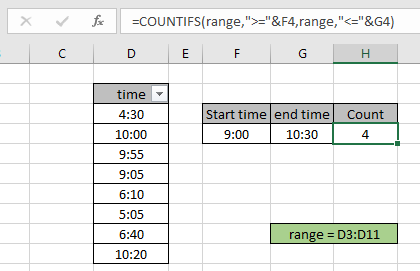While working with dates and times, we may get the need to count the time values between two given time values. In this article, we will see how can we use COUNTIF function to count times between a given range.

How to solve the problem?

For this article we will be required to use the COUNTIFS function of course. If we are given time values in a range and specific two time values as criteria, we need to count the time values which lay between the two given times.

Generic formula:

= COUNTIFS ( range, ">="& sta_time, range, "<="& end_time )

Range : time values given in as range

Sta_time : start time value reference

End_time : end time value

Example: Count Time values between start time and end time inclusive

All of these might be confusing to understand. So, let's test this formula via counting times in an example.

Here we have the time records and we need to find the time values lying in between the given start and end time or return the count rows where the time value is between 9:00 to 10:30 time values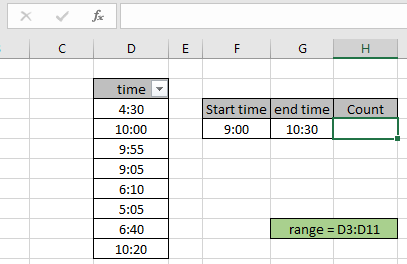Firstly, we need to define the named range for time range as range. Just select the time values and type the name (range) for the range in the top left corner as shown in the snapshot below..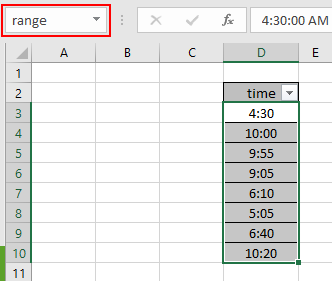Now we will use the following formula to get the count of times which lays in between 9:00 to 10:30 as given time values.
Use the Formula:

= COUNTIFS ( range , ">=" & F4 , range , "<=" & G4 )

range : named range used for birth date values D3:D11.

F4 : start time value given as cell reference.

G4 : end time value given as cell reference.

Explanation:

• COUNTIFS function counts the cells given multiple criteria
• Time values in excel operate with other time values
• The formula performs AND logic because it satisfies both conditions.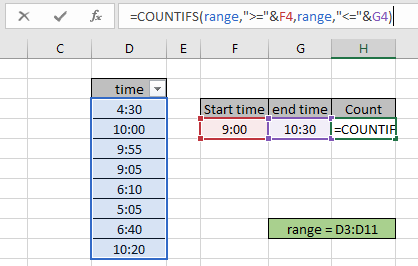Here the sta_time & end_time is given as cell reference and time range is given as cell reference. Press Enter to get the count.As you can see the total time values between 9:00 to 10:30 (including both) comes out to be 4.

You can check which time values lie between the range using the excel filter option. Apply the filter to the time header and click the arrow button which appears. Then select Number filters > Between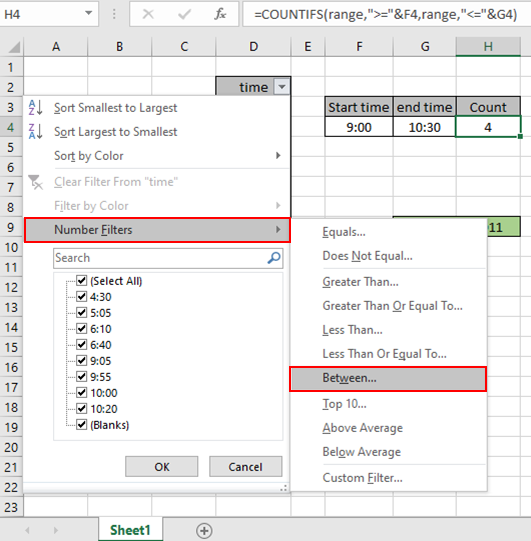A dialog box appears. Type the two time values and check other conditions also as shown below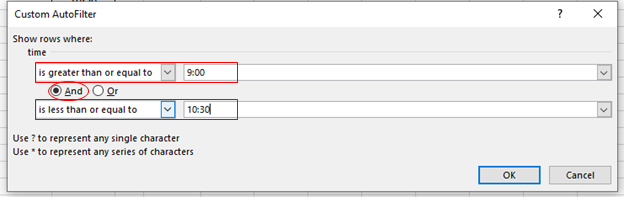After clicking OK, the time values will be filtered as shown below.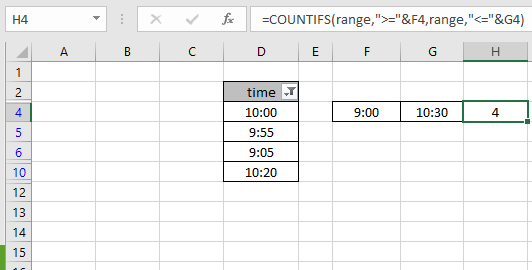As you can see all the 4 time values which lays between the given time values. This also means the formula works fine to get the count of these time values

Here are some observational notes shown below.

Notes:

1. The formula only works with numbers.
2. The argument array must be of the same length else the function returns error.
3. Operators like equals to ( = ), less than equal to ( <= ), greater than ( > ) or not equals to ( <> ) can be performed within function.

Hope this article about how to Count time values from range by month Excel is explanatory. Find more articles on COUNTIFS functions here.

If you liked our blogs, share it with your friends on Facebook. And also you can follow us on Twitter and Facebook. We would love to hear from you, do let us know how we can improve, complement or innovate our work and make it better for you. Write us at info@exceltip.com

Related Articles

How to use the SUMPRODUCT function in Excel: Returns the SUM after multiplication of values in multiple arrays in excel.

COUNTIFS with Dynamic Criteria Range : Count cells dependent on other cell values in Excel.

COUNTIFS Two Criteria Match : Count cells matching two different criteria on list in excel.

COUNTIFS With OR For Multiple Criteria : Count cells having multiple criteria match using the OR function.

The COUNTIFS Function in Excel : Count cells dependent on other cell values.

How to Use Countif in VBA in Microsoft Excel : Count cells using Visual Basic for Applications code.

How to use wildcards in excel : Count cells matching phrases using the wildcards in excel

Popular Articles

50 Excel Shortcut to Increase Your Productivity

Edit a dropdown list

Absolute reference in Excel

If with conditional formatting

If with wildcards

Vlookup by date

Terms and Conditions of use

The applications/code on this site are distributed as is and without warranties or liability. In no event shall the owner of the copyrights, or the authors of the applications/code be liable for any loss of profit, any problems or any damage resulting from the use or evaluation of the applications/code.